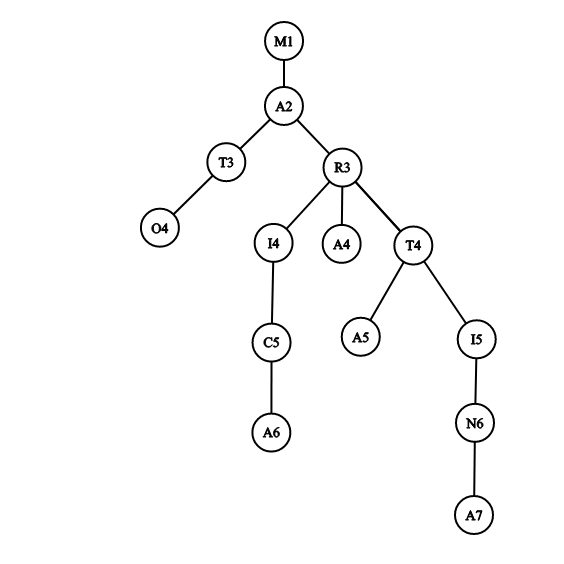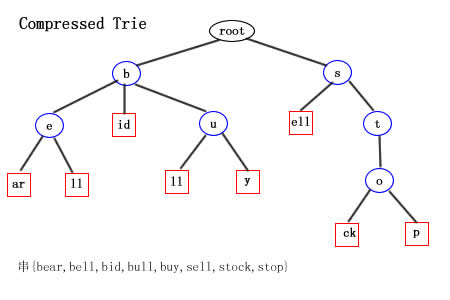# T1 AERODROM (二分答案

TimeLimit: 1000MS
Memory Limit: 32768KB

$N$个登机口，办理登机业务，第$i$个窗口的单位办理时间为$T_i$$M$个人办理登机业务，他们可以选择最佳的方案，不考虑换人和换窗口的时间，所有窗口是同时计时的，即同时开始办理业务，请输出所有人都登机的最少时间。

2个窗口，6个人，第一个窗口的单位时间是7,第二个是10, 一二个人分别在两个窗口办理，7秒时第三个人可在第一个窗口开始办理，10秒时，第四人开始在窗口二办理，时间14时，第五人一窗口。在时间20，窗口2可以使用，如果第六人在此办理，总时间将是30秒，如果等1秒在一窗口办理，则总时间是28秒。

input

2 6
7
10


output

28


input

7 10
3
8
3
6
9
2
4


output

8


$Code$

#include<bits/stdc++.h>
#define ll long long
using namespace std;
const int N=100005,M=1000000005;
int n,m;
int a[N],mins=0x7fffffff;
ll l,r;
{
int x=0,f=1;char st=getchar();
while(st<'0'||st>'9'){if(st=='-') f=-1;st=getchar();}
while(st>='0'&&st<='9') x=x*10+st-'0',st=getchar();
return x*f;
}

inline bool check(ll mid)
{
ll tmp=0;
for(int i=1;i<=n;i++)
{
tmp+=mid/a[i];
if(tmp>=m) return 1;
}
return 0;
}

int main()
{
freopen("aerodrom.in","r",stdin);
freopen("aerodrom.out","w",stdout);
for(int i=1;i<=n;i++)
{
mins=min(mins,a[i]);
}
r=(ll)mins*m;
while(l<r)
{
ll mid=l+r>>1;
if(check(mid)) r=mid;
else l=mid+1;
}
printf("%lld",l);
fclose(stdin);
fclose(stdout);
return 0;
}


# T2 HERKABE (trie

TimeLimit: 1000MS
Memory Limit: 32768KB

input

3
IVO
JASNA
JOSIPA


output

4


input

5
MARICA
MARTA
MATO
MARA
MARTINA


output

24


input

4
A
AA
AAA
AAAA


output

8• vector 代替字符集；
• 排序后模拟$trie$树；

$trie$树时间复杂度一样，这部分是$O(n^2)$;

$Code$

#include<bits/stdc++.h>
#define ll long long
using namespace std;
const int N=3005,mod=1000000007;
int n;
struct skr
{
char s[N];
int len;
bool operator<(skr w)const
{
for(int i=0;i<max(len,w.len);i++)
if(s[i]<w.s[i]) return 1;
else if(s[i]>w.s[i]) return 0;
}
}a[N];
ll ans=1,nm[N];
{
int x=0,f=1;char st=getchar();
while(st<'0'||st>'9'){if(st=='-') f=-1;st=getchar();}
while(st>='0'&&st<='9') x=x*10+st-'0',st=getchar();
return x*f;
}

inline void dfs(int x,int l,int r) //搜第x位，搜索区间是l~r
{
if(l>=r) return ;
int num=0;
int L=l,R=l;
for(int i=l;i<=r;i++)
{
if(a[i].s[x]==a[L].s[x]) R=i;
else
{
num++;
dfs(x+1,L,R);
L=i;
}
}
num++;
if(L==r) R=r;
dfs(x+1,L,R);
ans=ans*nm[num]%mod;
}

int main()
{
freopen("herkabe.in","r",stdin);
freopen("herkabe.out","w",stdout);
for(int i=1;i<=n;i++)
{
scanf("%s",a[i].s);
a[i].len=strlen(a[i].s);
a[i].s[a[i].len]='Z'+1;
a[i].len++;
}
nm=1;
for(int i=1;i<=n;i++)
nm[i]=nm[i-1]*i%mod;
sort(a+1,a+1+n);
dfs(0,1,n);
printf("%lld",ans);
fclose(stdin);
fclose(stdout);
return 0;
}

• 压缩$trie$

​ 比如这是一棵压缩了的$trie$树：（图片来自网络

# T2 HERKABE (扩展域并查集

TimeLimit: 1000MS
Memory Limit: 32768KB

input

3 3
2 1 2
1
2 1 3
2
2 2 3
3


output

0 1 2


input

4 6
2 4 2
3
2 4 1
6
1 3 1
2 3 1
2
1 2 2
2 2 3
7


output

5 0 14 3


input

5 6
2 4 2
10
2 5 3
2
2 2 3
1
2 1 4
3
1 3 1
2 3 4
2147483663


output

 15 6 7 12 5


$1$操作呢，我们发现它只是把我们的二进制串移了个位置，可以记录一个$r[i]$表示第$i$个串被右移了多少位；

$1$域同样；

$Code$

#include<bits/stdc++.h>
#define c n*32
#define ll long long
using namespace std;
const int N=100005;

int n,e,opt;
char ans[N];//卡内存
int r[N];
int fa[N*32*2],f1,f2;
ll sq,ra;

{
int x=0,f=1;char st=getchar();
while(st<'0'||st>'9'){if(st=='-') f=-1;st=getchar();}
while(st>='0'&&st<='9') x=x*10+st-'0',st=getchar();
return x*f;
}

inline int get(int x)
{
return fa[x]==x?x:fa[x]=get(fa[x]);
}

int main()
{
freopen("procesor.in","r",stdin);
freopen("procesor.out","w",stdout);

memset(fa,-1,sizeof fa);
for(int i=0;i<=n*32*2;i++)
fa[i]=i;
for(int i=1;i<=e;i++)
{
if(opt==1)
{
r[x]=(r[x]+y)%32;
}
else
{
scanf("%lld",&z);
for(int j=0;j<32;j++)
if((z>>j)&1)
{
int x1=(j+r[x])%32,x2=(j+r[y])%32;
f1=get((x-1)*32+x1),f2=get((y-1)*32+x2+c);
if(f1!=f2)
fa[f1]=f2;
f1=get((x-1)*32+x1+c),f2=get((y-1)*32+x2);
if(f1!=f2)
fa[f1]=f2;
}
else
{
int x1=(j+r[x])%32,x2=(j+r[y])%32;
f1=get((x-1)*32+x1),f2=get((y-1)*32+x2);
if(f1!=f2)
fa[f1]=f2;
f1=get((x-1)*32+x1+c),f2=get((y-1)*32+x2+c);
if(f1!=f2)
fa[f1]=f2;
}
}
}
for(int i=0;i<32*n;i++)
{
f1=get(i),f2=get(i+c);
if(f1==f2)
{
printf("-1");
fclose(stdin);
fclose(stdout);
return 0;
}
}
for(int j=1;j<=n;j++)
for(int i=0;i<32;i++)
ans[j][i]=2;//初始
for(int j=1;j<=n;j++)
for(int i=31;i>=0;i--)
{
bool fl1=0,fl2=0;
f1=get((j-1)*32+i);//0
f2=get((j-1)*32+i+c);//1
if(f1>=c) f1-=c,fl1=1;
if(f2>=c) f2-=c,fl2=1;
if(ans[(f1)/32+1][f1%32]==(fl1==1))
{
ans[j][i]=0;
}
else
{
if(ans[(f2)/32+1][f2%32]==(fl2==1))
ans[j][i]=1;
else
{
ans[j][i]=0;
ans[(f1)/32+1][f1%32]=fl1;
}
}
}
sq=1;
for(int i=1;i<=32;i++)
sq[i]=sq[i-1]*2;
for(int j=1;j<=n;j++)
{
ra=0;
for(int i=0;i<32;i++)
{
ra=ra+sq[i]*ans[j][i];
}
printf("%lld ",ra);
}

fclose(stdin);
fclose(stdout);
return 0;
}

posted @ 2019-12-09 17:37  YudeS楪  阅读(171)  评论(0编辑  收藏  举报